/ +91 9829211106
Welcome to Vidhyarthi Darpan

# Maths 12th Previous Year Question Paper 2019 (CBSE)

###### Physics 12th Previous Year Question Paper 2018 (CBSE)
November 30, 2019
###### Maths 12th Previous Year Question Paper 2019 (CBSE)
November 30, 2019MathsSET-I

Section – AQ.1. If A is a square matrix satisfying A’ A = I, write the value of |A|.

Solution: Given, A’ A= IQ.2. If y = x | x |, find for x &lt; 0.

Solution: If y = x | x |Q.3. Find the order and degree (if defined) of the differential equation

Solution:

Order of this equation is 2.

Degree of this equation is not defined.Q.4. Find the direction cosines of a line which makes equal angles with the coordinate axes.

Solution : Let the direction cosines of the line make an angle with each of the coordinate axes and direction cosines be l, m and n.

ORQ.4 A line passes through the point with position vector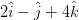and is in the direction of the vector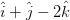. Find the equation of the line in cartesian form.

Solution: The line passes through a point (2, -1, 4) and has direction ratios proportional to (1, 1, – 2).

Cartesian equation of the line

Section – BQ.5. Examine whether the operation * defined on R, the set of all real numbers, by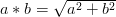is a binary operation or not, and if it is a  binary operation, find out whether it is associative or not.**Q.6. If A =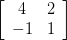, show that (A – 2I)(A – 3I) = 0.

Solution: Given,Q.7. Find

Solution:Q.8. Find

Solution:

ORQ.8. Find

Solution:Q.9. Find the differential equation of the family of curves y = Ac2x + Be-2x, where A and B are arbitrary constants.

Solution: Given, y = Ae2x + Be-2x         ………(i)

On differentiating equation (i) w.r.t. x, we getQ.10. If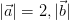and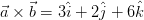, find the angle between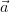and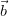.

Solution: Given,

ORQ.10. Find the volume of a cuboid whose edges are given by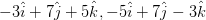and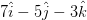.

Solution: If a, b, c are edges of a cuboid.Q.11. If P(not A) = 0·7, P(B) = 0·7 and P(B/A) = 0·5, then find P(A∆B).

Solution:Q.12. A coin is tossed 5 times. What is the probability of getting (i) 3 heads, (ii) at most 3 heads?

Solution:

ORQ.12. Find the probability distribution of X, the number of heads in a simultaneous toss of two coins.

Solution: If we toss two coins simultaneously then sample space is given by (HH, HT, TH, IT)

Then probability distribution is,

Section – CQ.13. Check whether the relation R defined on the set A = {1, 2, 3, 4, 5, 6} as R = {(a, b) : b = a + 1} is reflexive, symmetric or transitive.

Solution: Here, R = {(a, b): b = a +1}

∴ R = {(a, a + 1): a, a + 1 ϵ (1, 2, 3, 4, 5 ,6)}

⇒ R = {(1, 2,) (2, 3), (3, 4), (4, 5), (5, 6)}

(i) R is not reflexive as {a, a} ∉ R∀a

(ii) R is not symmetric as (1, 2) ϵ R but (2, 1) ∉ R

(iii) R is not transitive as (1, 2) ϵ R, (2, 3) ϵ R but (1, 3) ϵ R

ORQ.13. Let f: N → Y be a function defined as

f(x) = 4x + 3,

where Y = {y ϵ N : y = 4x + 3, for some x ϵ N}.

Show that f is invertible. Find its inverse.

Solution: Consider an arbitrary element of Y. By the definition of y, y = 4x + 3, for some x in the domain N.Q.14. Find the value

Solution: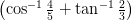Q.15. Using properties of determinants, show that

Solution :Q.16. If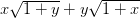= 0 and x ≠ y, prove that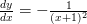Solution:

Solution:Q.17. If If (x – a)2 + (y – b)2 = c2, for some c > 0, prove that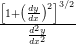is a constant independent of a and b.

Solution:Q.18. Find the equation of the normal to the curve x2 = 4y which passes through the point (-1, 4).

Solution: Suppose the normal at P(x1, y1) on the parabola x 1 = 4y passes through (-1, 4)

Since, P(x1, y1) lies on x1 = 4y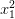= 4y1

The equation of curve is x2 = 4y.

Differentiating with respect to x, we have

2x = 4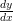Q.19. Find

Solution:Q.20. Prove that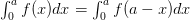and hence evaluate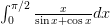.

Solution:Q.21. Solve the differential equation:

Solution:Q.22. The scalar product of the vector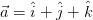with a unit vector along the sum of the vectors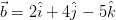and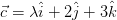is equal to 1. Find the value of λ and hence find the unit vector along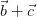.

Solution:Q.23. If the lines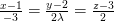and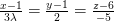are perpendicular, find the value of λ. Hence find whether the lines are intersecting or not.

Solution:

Section – DQ.24. If A =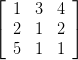, Find A-1.

Hence solve the system of equations

x + 3y + 4z = 8

2x + y + 2z = 5

and 5x + y + z = 7

Solution :

ORQ.24. Find the inverse of the following matrix, using elementary transformation:

A =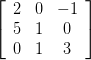Solution:Q.25. Show that the height of the cylinder of maximum volume that can be inscribed in a sphere of radius R is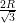. Also find the maximum volume.

Solution: Let, ‘x’ be the diameter of the base of the cylinder and let ‘h’ be the height of the cylinder.Q.26. Using method of integration, find the area of the triangle whose vertices are (1, 0), (2, 2) and (3, 1).

Solution: A(1, 0), B(2, 2) and C(3, 1)

OR

Q.26. Using method of integration, find the area of the region enclosed between two circles x2 + y2 = 4 and (x – 2)2 + y2 = 4.

Solution: Equations of the given circles are,

x2 + y2 = 4 …(i)

(x – 2)2 + y2 = 4 …(ii)

Equation (i) is a circle with centre O at the origin and radius 2. Equation (ii) is a circle with centre C (2, 0) and radius 2.

Solving equation (i) and (ii) we have

(x – 2)2 + y2 = x2 + y2

or x2 – 4x + 4 + y2 = x2 + y2 or x = 1 which gives y = ±

Thus, the points of intersection of the given circles are A (1, 3) and A (1, 3)Q.27. Find the vector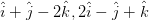and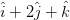cartesian equations of the plane passing through the points having position vectors and . Write the equation of a plane passing through a point (2, 3, 7) and parallel to the plane obtained above. Hence, find the distance between the two parallel planes.

Solution: Let A, B, C be the points with position

ORQ.27. Find the equation of the line passing through (2, -1, 2) and (5, 3, 4) and of the plane passing through (2, 0, 3), (1, 1, 5) and (3, 2, 4). Also, find their point of intersection.

Solution: We know that the equation of a line passing through points (x1, y1, z1,) and (x2, y2, z2) ) is given byQ.28. There are three coins. One is a two-headed coin, another is a biased coin that comes up heads 75% of the time and the third is an unbiased coin. One of the three coins is chosen at random and tossed. If it shows heads, what is the probability that it is the two-headed coin?

Solution: Given, there are three coins.

Let,

E1 = coin is two headed

E2 = biased coin

E3 = unbiased coin

A = shows only headQ.29. A company produces two types of goods, A and B, that require gold and silver. Each unit of type A requires 3g of silver and 1 g of gold while that of type B requires 1 g of silver and 2g of gold. The company can use at most 9 g of silver and 8 of gold. If each unit of type A brings a profit of ₹ 40 and that of type B ₹ 50, find the number of units of each type that the company should produce to maximize profit. Formulate the above LPP and solve it graphically and also find the maximum profit.

Solution: There are two types of goods, A and B and let units of type A be x and units of type B be y.

Next Paper SET-II and SET-III Click Here!×

### Hello!

Click one of our representatives below to chat on WhatsApp or send us an email to info@vidhyarthidarpan.com

×